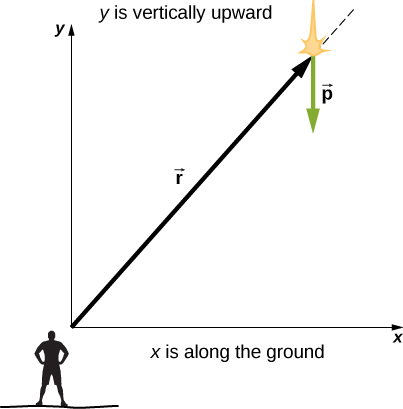# 11.2 Angular momentum  (Page 2/8)

 Page 2 / 8

Here we have used the definition of $\stackrel{\to }{p}$ and the fact that a vector crossed into itself is zero. From Newton’s second law, $\frac{d\stackrel{\to }{p}}{dt}=\sum \stackrel{\to }{F},$ the net force acting on the particle, and the definition of the net torque, we can write

$\frac{d\stackrel{\to }{l}}{dt}=\sum \stackrel{\to }{\tau }.$

Note the similarity with the linear result of Newton’s second law, $\frac{d\stackrel{\to }{p}}{dt}=\sum \stackrel{\to }{F}$ . The following problem-solving strategy can serve as a guideline for calculating the angular momentum of a particle.

## Problem-solving strategy: angular momentum of a particle

1. Choose a coordinate system about which the angular momentum is to be calculated.
2. Write down the radius vector to the point particle in unit vector notation.
3. Write the linear momentum vector of the particle in unit vector notation.
4. Take the cross product $\stackrel{\to }{l}=\stackrel{\to }{r}\phantom{\rule{0.2em}{0ex}}×\phantom{\rule{0.2em}{0ex}}\stackrel{\to }{p}$ and use the right-hand rule to establish the direction of the angular momentum vector.
5. See if there is a time dependence in the expression of the angular momentum vector. If there is, then a torque exists about the origin, and use $\frac{d\stackrel{\to }{l}}{dt}=\sum \stackrel{\to }{\tau }$ to calculate the torque. If there is no time dependence in the expression for the angular momentum, then the net torque is zero.

## Angular momentum and torque on a meteor

A meteor enters Earth’s atmosphere ( [link] ) and is observed by someone on the ground before it burns up in the atmosphere. The vector $\stackrel{\to }{r}=25\phantom{\rule{0.2em}{0ex}}\text{km}\stackrel{^}{i}+25\phantom{\rule{0.2em}{0ex}}\text{km}\stackrel{^}{j}$ gives the position of the meteor with respect to the observer. At the instant the observer sees the meteor, it has linear momentum $\stackrel{\to }{p}=15.0\phantom{\rule{0.2em}{0ex}}\text{kg}\left(-2.0\text{km}\text{/}\text{s}\stackrel{^}{j}\right)$ , and it is accelerating at a constant $2.0\phantom{\rule{0.2em}{0ex}}\text{m}\text{/}{\text{s}}^{2}\left(\text{−}\stackrel{^}{j}\right)$ along its path, which for our purposes can be taken as a straight line. (a) What is the angular momentum of the meteor about the origin, which is at the location of the observer? (b) What is the torque on the meteor about the origin?An observer on the ground sees a meteor at position r → with linear momentum p → .

## Strategy

We resolve the acceleration into x - and y -components and use the kinematic equations to express the velocity as a function of acceleration and time. We insert these expressions into the linear momentum and then calculate the angular momentum using the cross-product. Since the position and momentum vectors are in the xy -plane, we expect the angular momentum vector to be along the z -axis. To find the torque, we take the time derivative of the angular momentum.

## Solution

The meteor is entering Earth’s atmosphere at an angle of $90.0\text{°}$ below the horizontal, so the components of the acceleration in the x - and y -directions are

${a}_{x}=0,\phantom{\rule{0.5em}{0ex}}{a}_{y}=-2.0\phantom{\rule{0.2em}{0ex}}\text{m}\text{/}{\text{s}}^{2}.$

We write the velocities using the kinematic equations.

${v}_{x}=0,\phantom{\rule{0.5em}{0ex}}{v}_{y}=-2.0\phantom{\rule{0.2em}{0ex}}×\phantom{\rule{0.2em}{0ex}}{10}^{3}\phantom{\rule{0.2em}{0ex}}\text{m}\text{/}\text{s}-\left(2.0\phantom{\rule{0.2em}{0ex}}\text{m}\text{/}{\text{s}}^{2}\right)t.$
1. The angular momentum is
$\begin{array}{cc}\hfill \stackrel{\to }{l}& =\stackrel{\to }{r}\phantom{\rule{0.2em}{0ex}}×\phantom{\rule{0.2em}{0ex}}\stackrel{\to }{p}=\left(25.0\phantom{\rule{0.2em}{0ex}}\text{km}\stackrel{^}{i}+25.0\phantom{\rule{0.2em}{0ex}}\text{km}\stackrel{^}{j}\right)\phantom{\rule{0.2em}{0ex}}×\phantom{\rule{0.2em}{0ex}}15.0\phantom{\rule{0.2em}{0ex}}\text{kg}\left(0\stackrel{^}{i}+{v}_{y}\stackrel{^}{j}\right)\hfill \\ & =15.0\phantom{\rule{0.2em}{0ex}}\text{kg}\left[25.0\phantom{\rule{0.2em}{0ex}}\text{km}\left({v}_{y}\right)\stackrel{^}{k}\right]\hfill \\ & =15.0\phantom{\rule{0.2em}{0ex}}\text{kg[}2.50\phantom{\rule{0.2em}{0ex}}×\phantom{\rule{0.2em}{0ex}}{10}^{4}\phantom{\rule{0.2em}{0ex}}\text{m}\left(-2.0\phantom{\rule{0.2em}{0ex}}×\phantom{\rule{0.2em}{0ex}}{10}^{3}\phantom{\rule{0.2em}{0ex}}\text{m}\text{/}\text{s}-\left(2.0\phantom{\rule{0.2em}{0ex}}\text{m}\text{/}{\text{s}}^{2}\right)t\right)\stackrel{^}{k}\right].\hfill \end{array}$

At $t=0$ , the angular momentum of the meteor about the origin is
${\stackrel{\to }{l}}_{0}=15.0\phantom{\rule{0.2em}{0ex}}\text{kg}\left[2.50\phantom{\rule{0.2em}{0ex}}×\phantom{\rule{0.2em}{0ex}}{10}^{4}\phantom{\rule{0.2em}{0ex}}\text{m}\left(-2.0\phantom{\rule{0.2em}{0ex}}×\phantom{\rule{0.2em}{0ex}}{10}^{3}\phantom{\rule{0.2em}{0ex}}\text{m}\text{/}\text{s}\right)\stackrel{^}{k}\right]=7.50\phantom{\rule{0.2em}{0ex}}×\phantom{\rule{0.2em}{0ex}}{10}^{8}\phantom{\rule{0.2em}{0ex}}\text{kg}·{\text{m}}^{2}\text{/}\text{s}\left(\text{−}\stackrel{^}{k}\right).$

This is the instant that the observer sees the meteor.
2. To find the torque, we take the time derivative of the angular momentum. Taking the time derivative of $\stackrel{\to }{l}$ as a function of time, which is the second equation immediately above, we have
$\frac{d\stackrel{\to }{l}}{dt}=-15.0\phantom{\rule{0.2em}{0ex}}\text{kg}\left(2.50\phantom{\rule{0.2em}{0ex}}×\phantom{\rule{0.2em}{0ex}}{10}^{4}\phantom{\rule{0.2em}{0ex}}\text{m}\right)\left(2.0\phantom{\rule{0.2em}{0ex}}\text{m}\text{/}{\text{s}}^{2}\right)\stackrel{^}{k}.$

Then, since $\frac{d\stackrel{\to }{l}}{dt}=\sum \stackrel{\to }{\tau }$ , we have
$\sum \stackrel{\to }{\tau }=-7.5\phantom{\rule{0.2em}{0ex}}×\phantom{\rule{0.2em}{0ex}}{10}^{5}\text{N}·\text{m}\stackrel{^}{k}.$

The units of torque are given as newton-meters, not to be confused with joules. As a check, we note that the lever arm is the x -component of the vector $\stackrel{\to }{\text{r}}$ in [link] since it is perpendicular to the force acting on the meteor, which is along its path. By Newton’s second law, this force is
$\stackrel{\to }{F}=ma\left(\text{−}\stackrel{^}{j}\right)=15.0\phantom{\rule{0.2em}{0ex}}\text{kg}\left(2.0\phantom{\rule{0.2em}{0ex}}\text{m}\text{/}{\text{s}}^{2}\right)\left(\text{−}\stackrel{^}{j}\right)=30.0\phantom{\rule{0.2em}{0ex}}\text{kg}·\text{m}\text{/}{\text{s}}^{2}\left(\text{−}\stackrel{^}{j}\right).$

The lever arm is
${\stackrel{\to }{r}}_{\perp }=2.5\phantom{\rule{0.2em}{0ex}}×\phantom{\rule{0.2em}{0ex}}{10}^{4}\phantom{\rule{0.2em}{0ex}}\text{m}\phantom{\rule{0.2em}{0ex}}\stackrel{^}{i}.$

Thus, the torque is
$\begin{array}{cc}\hfill \sum \stackrel{\to }{\tau }={\stackrel{\to }{r}}_{\perp }\phantom{\rule{0.2em}{0ex}}×\phantom{\rule{0.2em}{0ex}}\stackrel{\to }{F}& =\left(2.5\phantom{\rule{0.2em}{0ex}}×\phantom{\rule{0.2em}{0ex}}{10}^{4}\phantom{\rule{0.2em}{0ex}}\text{m}\phantom{\rule{0.2em}{0ex}}\stackrel{^}{i}\right)\phantom{\rule{0.2em}{0ex}}×\phantom{\rule{0.2em}{0ex}}\left(-30.0\phantom{\rule{0.2em}{0ex}}\text{kg}·\text{m}\text{/}{\text{s}}^{2}\stackrel{^}{j}\right),\hfill \\ & =7.5\phantom{\rule{0.2em}{0ex}}×\phantom{\rule{0.2em}{0ex}}{10}^{5}\phantom{\rule{0.2em}{0ex}}\text{N}·\text{m}\left(\text{−}\stackrel{^}{k}\right).\hfill \end{array}$

a length of copper wire was measured to be 50m with an uncertainty of 1cm, the thickness of the wire was measured to be 1mm with an uncertainty of 0.01mm, using a micrometer screw gauge, calculate the of copper wire used
Mustapha
If centripetal force is directed towards the center,why do you feel that you're thrown away from the center as a car goes around a curve? Explain
Which kind of wave does wind form
calculate the distance you will travel if you mantain an average speed of 10N m/s for 40 second
hw to calculate the momentum of the 2000.0 elephant change hunter at a speed of 7.50 m/s
how many cm makes 1 inches
2.5
omwoyo
2.54cm=1inche
omwoyo
how do we convert from m/s to km/hr
When paddling a canoe upstream, it is wisest to travel as near to the shore as possible. When canoeing downstream, it may be best to stay near the middle. Explain why?
Explain why polarization does not occur in sound
one ship sailing east with a speed of 7.5m/s passes a certain point at 8am and a second ship sailing north at the same speed passed the same point at 9.30am at what distance are they closet together and what is the distance between them then
density of a subtance is given as 360g/cm,put it in it s.i unit form
if m2 is twice of m1. find the ration of kinetic energy in COM system to lab system of elastic collision
What is a volt equal to?
list and explain the 3 ways of charging a conductor
conduction convention rubbing
Asdesaw
formula of magnetic field
why polarization does not occur in sound
Integral of a vector
define surface integral of a vector?
Rahat

#### Get Jobilize Job Search Mobile App in your pocket Now!By Mistry BhaveshBy P. Wynn NormanBy Rohini AjayBy Rohini AjayBy OpenStaxBy OpenStaxBy Brooke DelaneyBy OpenStaxBy Anh DaoBy Savannah Parrish